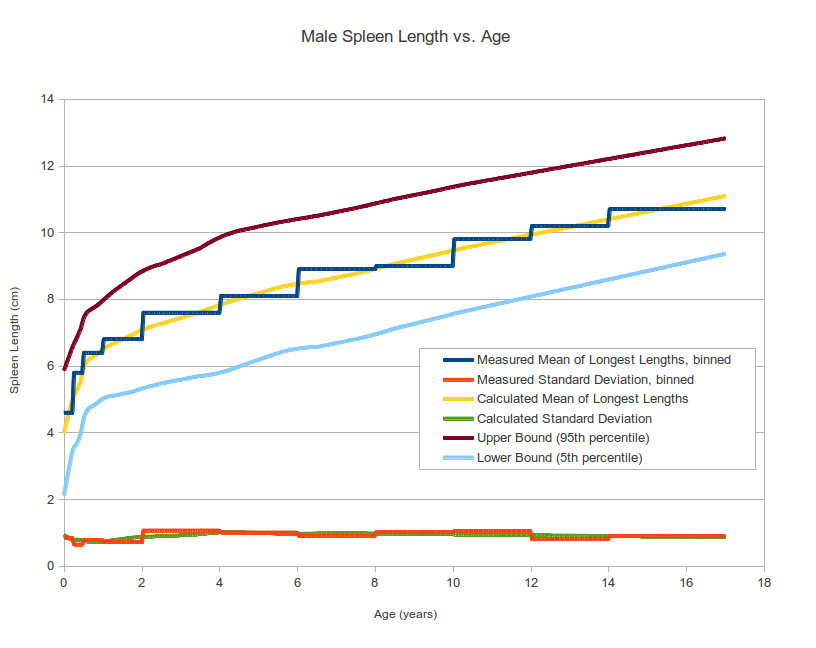# Pediatric Spleen Size Percentile Calculator

contributed by Michal Kulon, MD on 9/15/2015
 Year Month Day Date of Scan: Date of Birth: Spleen Length: cm Calculate Percentile:
For males aged 0 years 0 months 0 days, the average spleen length is 4 cm and standard deviation is 0.93 cm.
Spleen length of 0 cm corresponds to 0 percentile (4.28 standard deviations below the mean).## Methods

• Normal range data based on "Spleen Length in Childhood with US: Normal Values Based on Age, Sex, and Somatometric Parameters", Radiology April 2004 231:1 129-134
• Source data of normal spleen length consists of 454 children under 17 years old (249 females, 205 males). However, in the source article, this population is divided into numerous age intervals, with each interval containing relatively few patients (6 to 36). This yields good overall regression, but somewhat poorer statistical quality within some of the individual age intervals.
• This calculator performs multiple linear regressions, each linear regression centered at the age of interest, spanning relatively short age range. Exceptions are made for youngest and oldest age groups, where it not possible to center the linear regression, and also for ages under 3 years old, where linear regression over several years is not appropriate due to the curvature of the data for this age range. In calculating regression, higher weight is given to the age interval containing more patients.
• Calculation of length percentiles assumes a non-skewed, approximately normal distribution, and that the mean approximately equals the median.